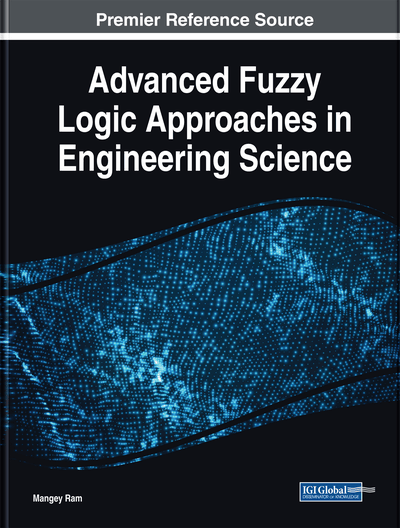# Reliability Analysis for Environment Systems Using Dual Hesitant Fuzzy Set

Akshay Kumar, Mangey Ram
DOI: 10.4018/978-1-5225-5709-8.ch008
OnDemand:
(Individual Chapters)
Available
\$37.50
No Current Special Offers

## Abstract

In this chapter, we deal with dual hesitant fuzzy set theory and compute the fuzzy reliability with lifetime components of different electronic systems, such as series and parallel systems from a Markov chain technique. In dual hesitant fuzzy sets, we have membership and non-membership degree function whereas hesitant fuzzy sets only have membership function. In this chapter we also discuss the Weibull distribution and reliability function of the proposed systems. A numerical example is also given in the end of proposed algorithm.
Chapter Preview
Top

## Introduction

In previous years fuzzy set theory, intuitionistic fuzzy, hesitant fuzzy and dual hesitant fuzzy is most important tool to evaluate the fuzzy reliability of different engineering systems. Reliability of the systems can be evaluated in different methods and techniques, but fuzzy reliability measures the uncertainty of the possible membership and possible non-membership grade of the components. Application of fuzzy reliability widely used in engineering fields such as decision making, correlation coefficient and distance measures etc. Zadeh (1965) first introduced the concept of fuzzy set theory which is based on the membership function. He discussed the various techniques for comparing fuzzy set theory and set theory on the basis of crisp sets. Atanassov (1983) also introduced the new concept of fuzzy set theory which is known as intuitionistic fuzzy set theory and this theory based on the membership degree function only. Hesitant fuzzy set (HFS) first introduced by Torra and Narukawa (2009) defined the concept given the extended form of the fuzzy set theory. They discussed possible values of membership function and employed the aggregation operators to find fuzzy possible values. Zhu et al. (2012) discussed the method of dual hesitant fuzzy set (DHFS) and also investigates the fuzzy set, intuitionistic fuzzy set and HFS on the basis of possible membership degree and non-membership degree functions. They measured the basic operations and properties of DHFS. Wang et al. (2014) investigated the various dual hesitant fuzzy aggregations operations such as dual hesitant fuzzy weighted average (DHFWA) operator, dual hesitant fuzzy weighted geometric (DHFWG) operator, and dual hesitant fuzzy ordered weighted average (DHFOWA) operator. Ye (2014) computed the correlation and correlation coefficient by using dual hesitant fuzzy membership and non-membership degree of two possible sets. Xu et al. (2015) described the nature of membership and non-membership function by using some aggregations operators and they discussed application of DHFS in the fields of data mining, clustering and medical diagnosis etc. Chen and Huang (2017) investigated the extended properties of dual hesitant fuzzy set theory with the help of dual hesitant fuzzy numbers in place of intuitionistic fuzzy set. They discussed the concept of dual hesitant fuzzy probability, dual hesitant fuzzy total probability and dual hesitant fuzzy conditional probability.

In the context of fuzzy reliability, in previous years many researchers elaborated the studies of fuzzy reliability by using various fuzzy like simple fuzzy, intuitionistic fuzzy, bifuzzy and hesitant fuzzy with membership and non-membership of possible degree functions. Sharma et al. (2012) computed the fuzzy reliability of the different system using intuitionistic fuzzy set. They implement the intuitionistic triangular fuzzy number and its arithmetic operations. Jamkhaneh (2014) evaluated the fuzzy reliability with help of Weibull distribution and fuzzy triangular number. The author investigated fuzzy reliability and mean time to failure series and parallel system. Kumar and Singh (2014) determined the fuzzy reliability of the network system using rough intuitionistic fuzzy set and discussed the different fuzzy reliability of the various environment systems. Kumar and Singh (2015) investigated the fuzzy reliability of series, parallel, series-parallel and parallel-series system from intuitionistic fuzzy using Weibull lifetime distribution. They evaluated the reliability function with the help of the membership and non-membership degree of triangular fuzzy numbers. Chaube and Singh (2016a, 2016b) evaluated the fuzzy reliability of various systems such as two states weighted k-out-of-n system and complex system from generalized minimal cuts and minimal paths. Kumar et al. (2016, 2017) determine the fuzzy reliability of different systems from using intuitionistic and hesitant fuzzy set. They discussed the membership function in case of hesitant fuzzy number and in case of intuitionistic fuzzy set both find membership and non-membership functions.

## Complete Chapter List

Search this Book:
Reset